After I moved to Victoria, I met someone who asked me to help him to build a quality assurance station to weigh the end product and kick out if the weight of the product is not in between the desired range. I wasn’t sure if I would be able to build it but I still accepted the task.

As usual I started with an arduino board and researched about the weigh sensors. At first, I wanted to use an arduino pro mini, but for easier programming purposes, I decided to use an arduino nano. Here is how it should work:

• We get the weight from the load sensor
• Then we convert the weight to voltage. We convert the information to voltage, because everything else is going to be handled by the PCB.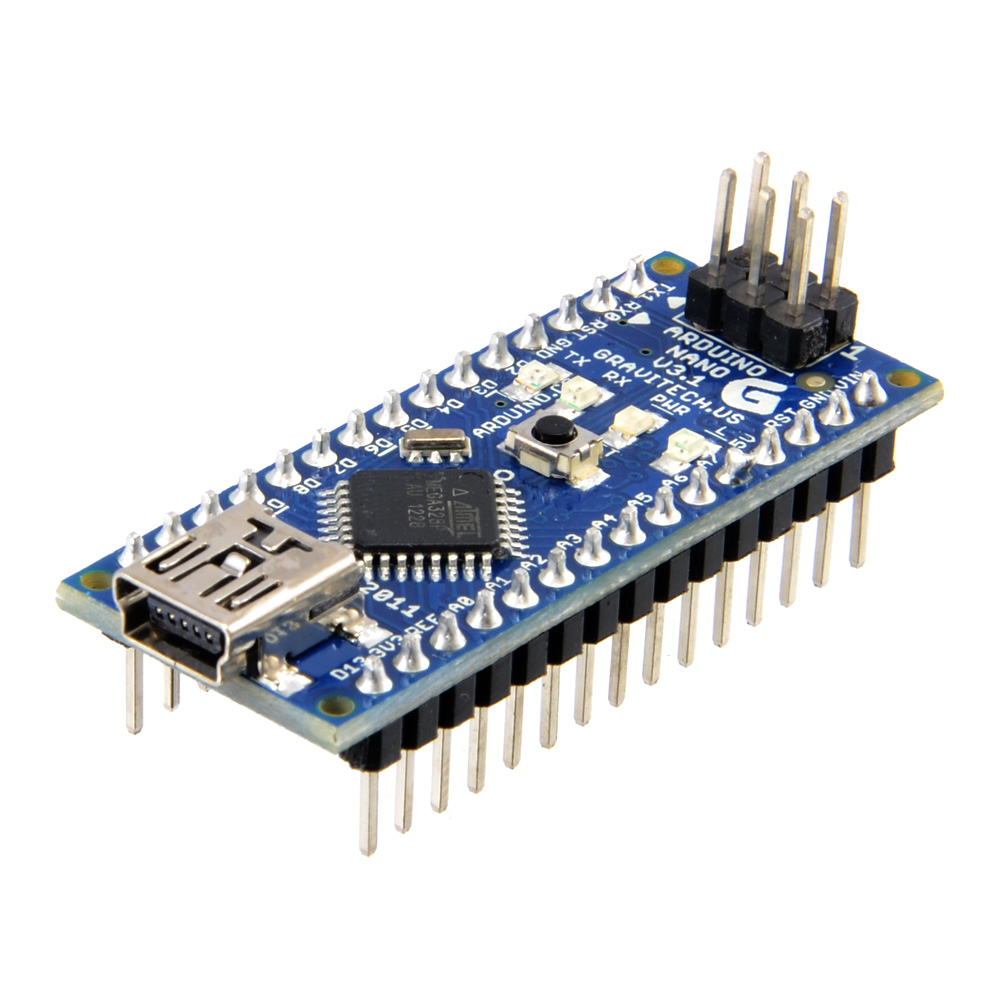There were not many options for a weigh sensor, so I ended up using the “Digital Load Cell Weight Sensor HX711 AD Converter”.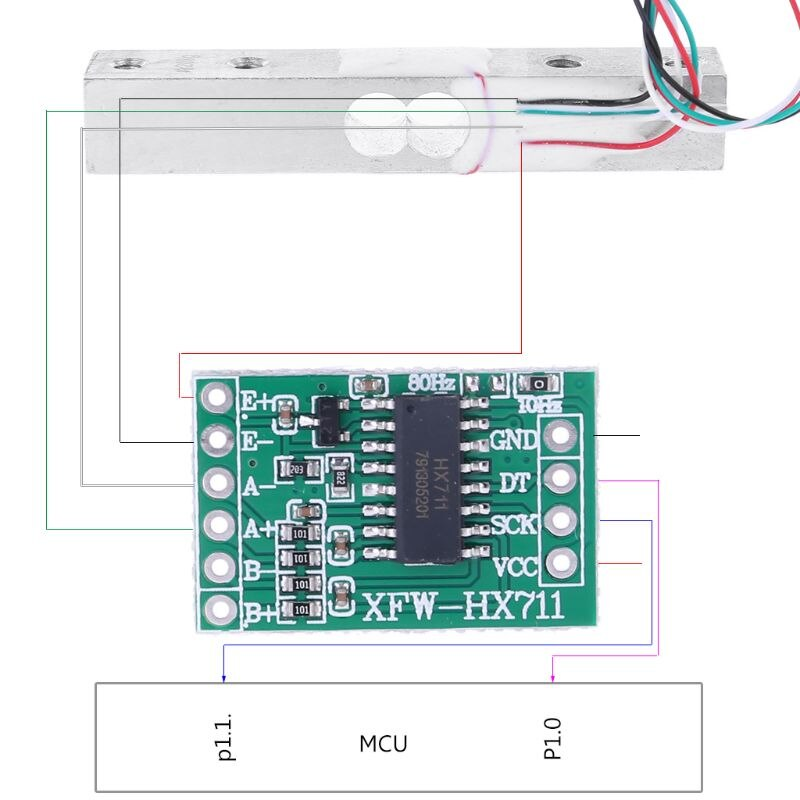I simply installed the HX711 library from the Arduino IDE library, yo ucan see it’s details here: bogde/HX711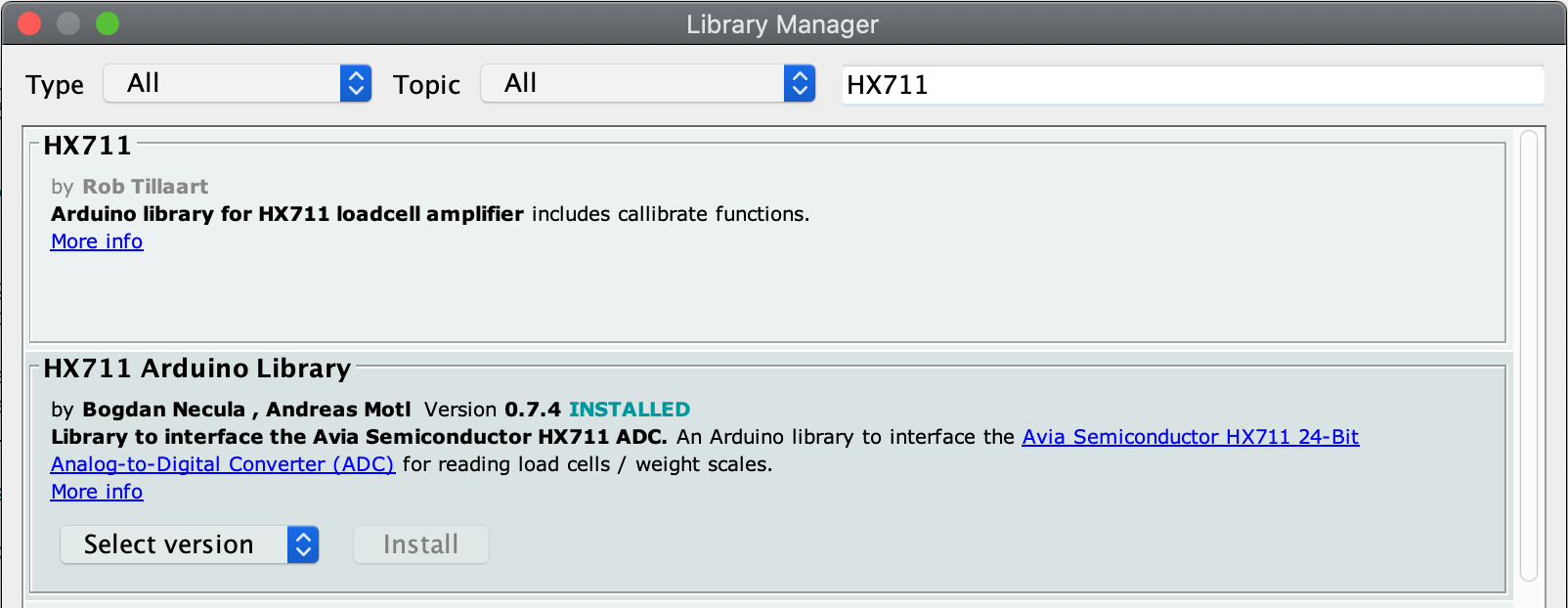Since the system’s main controller was a PLC, I was asked to convert the weight to a voltage range. So I found MCP4822 chip which was a great choice to output a voltage in between 0-4096 mV. The rest was handled by the PLC programming.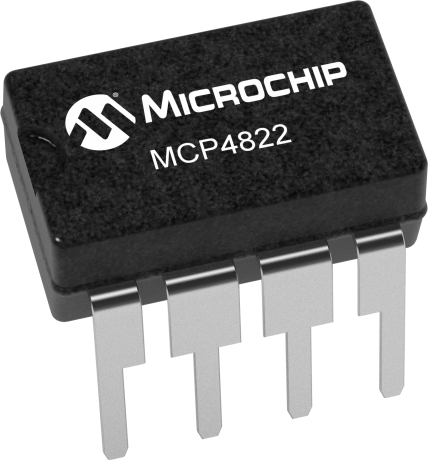I found this example online for the voltage convertor, it explained every detail about the process, but I simply used their code, thanks to mr. Mohamad.

I used Fritzing for the development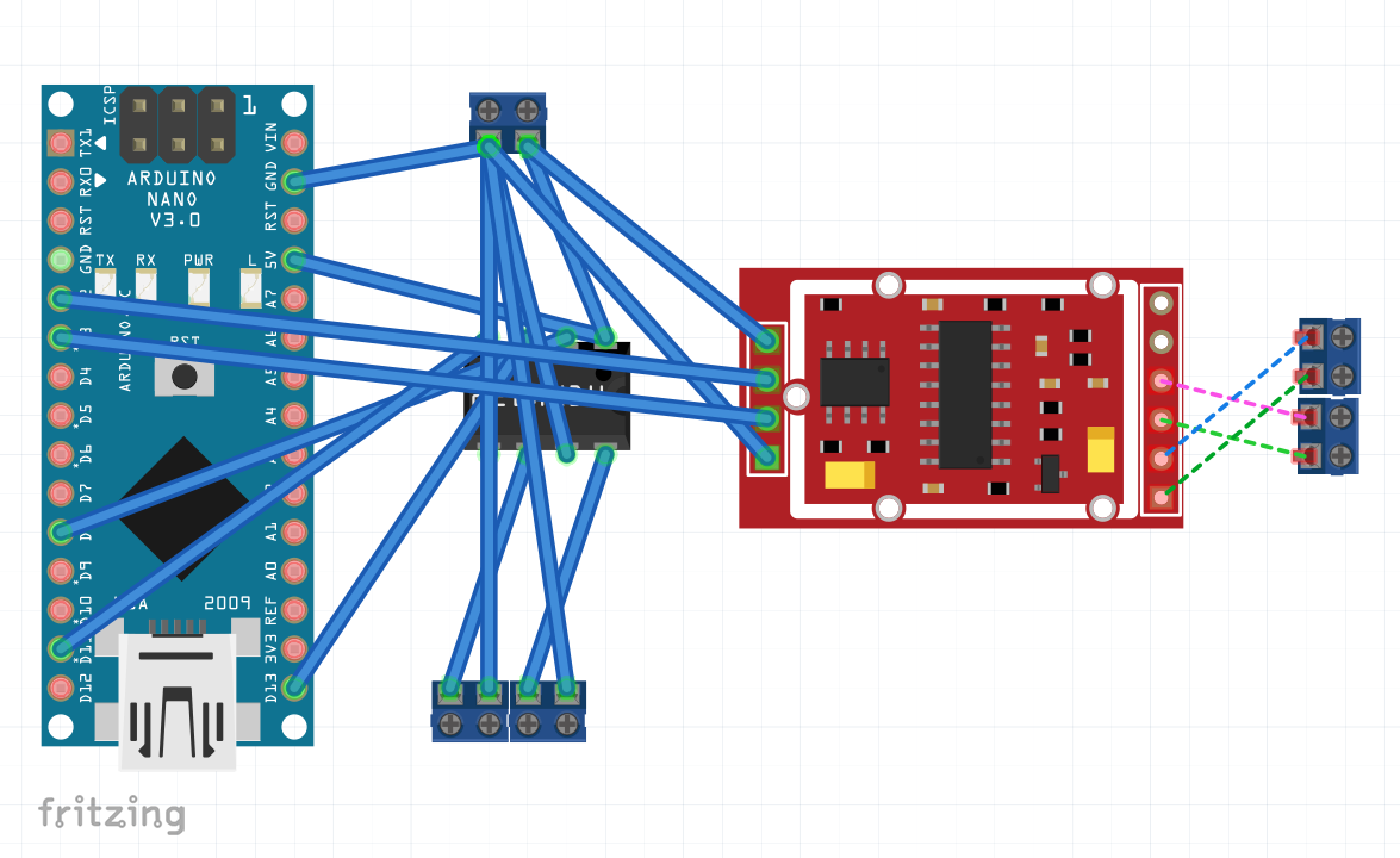Then I soldered them up on a simple board manually. And of course built a simple box with OpenSCAD and printed it out. This was also a good opportunity for me to test the Resin Printer I recently got.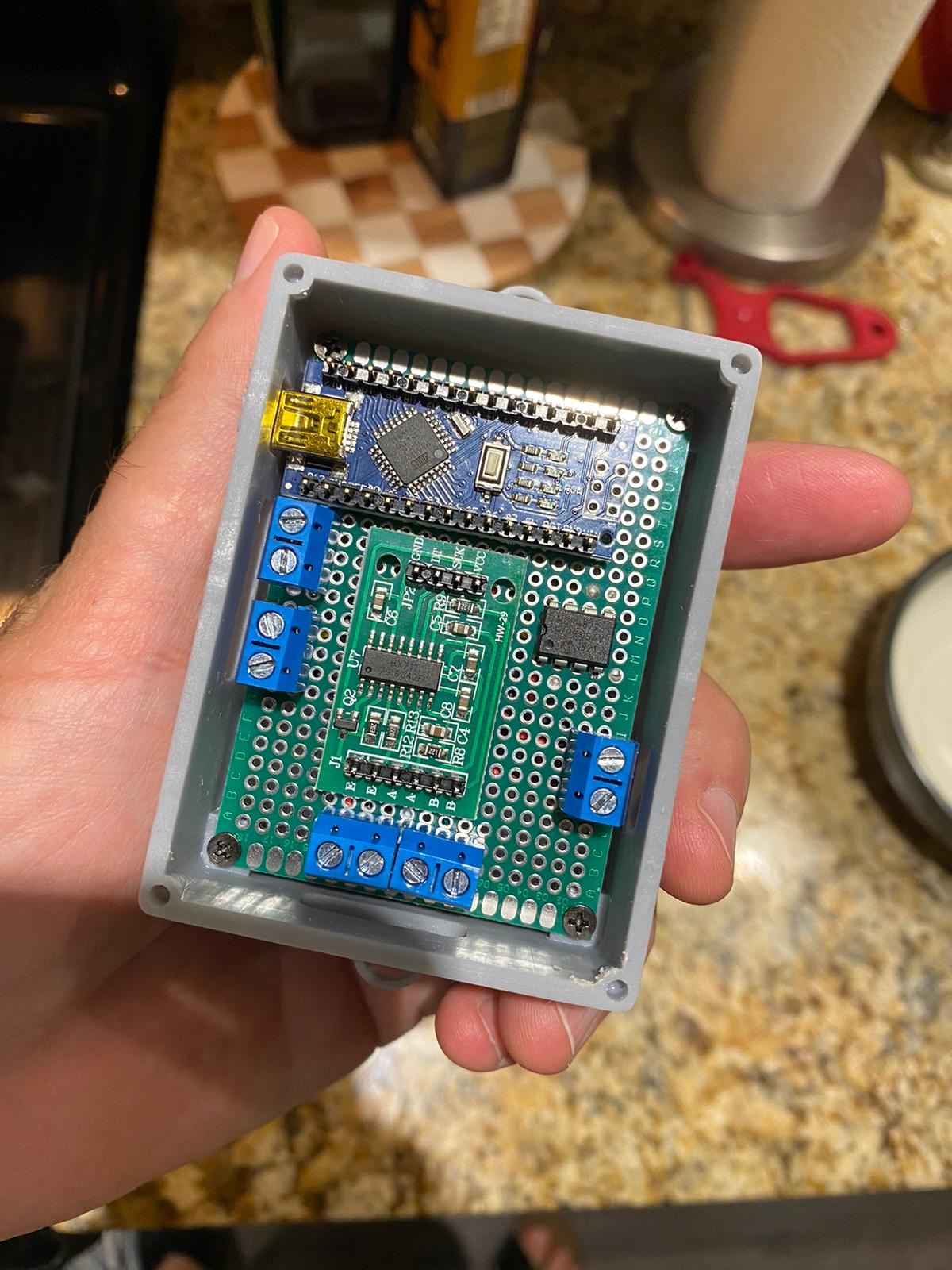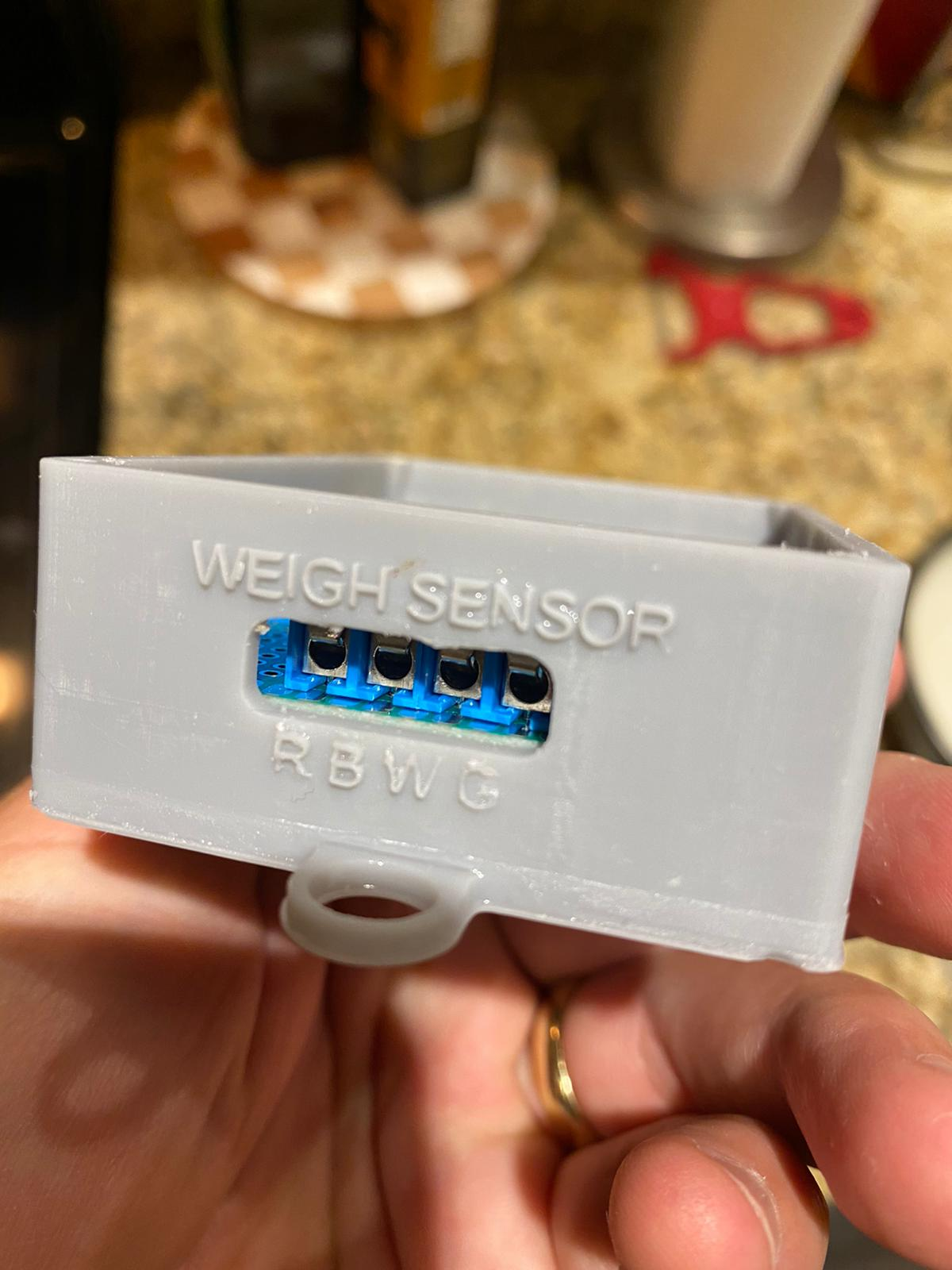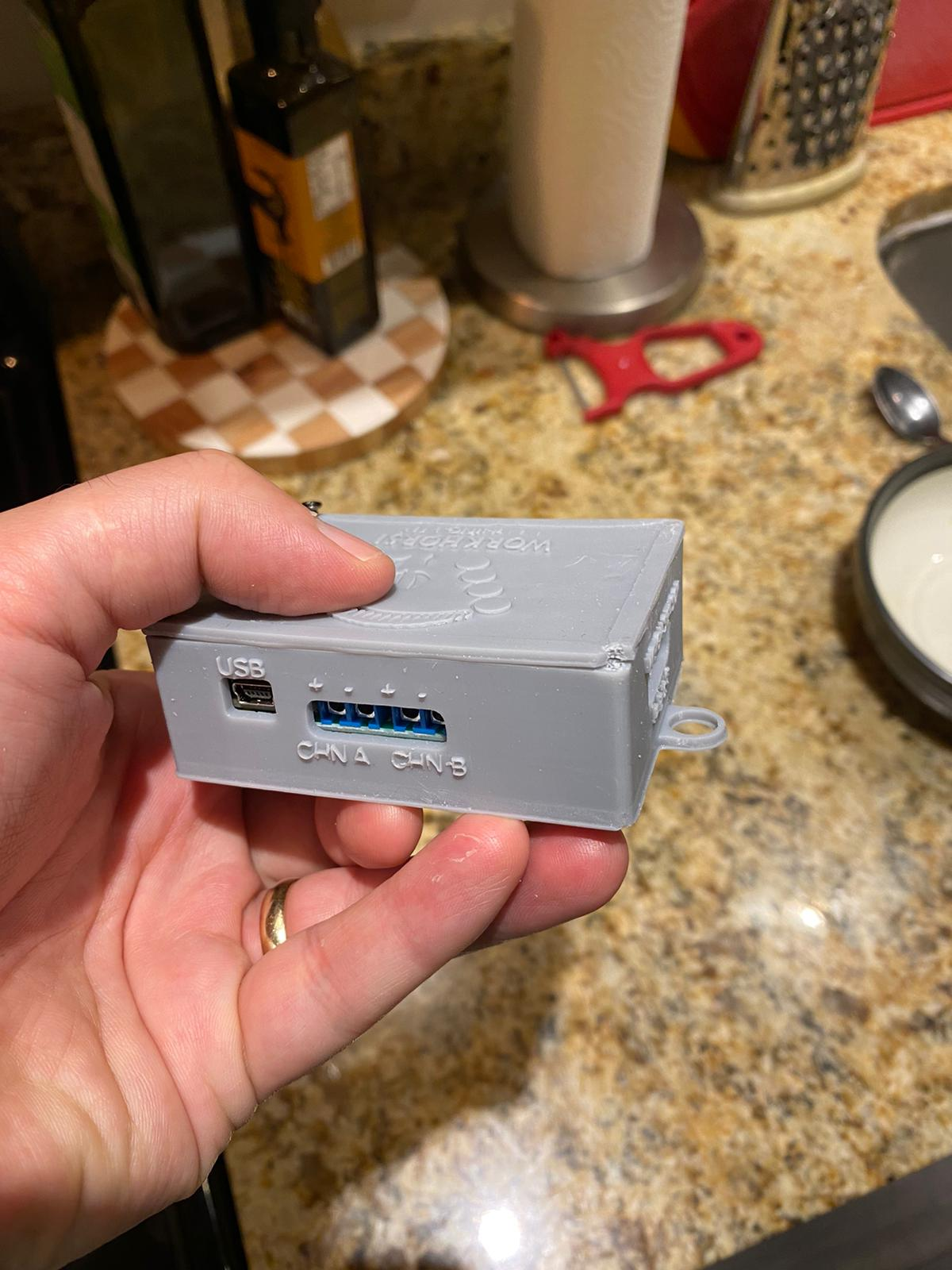We tested on the site and it was successful. So I decided to get the PCB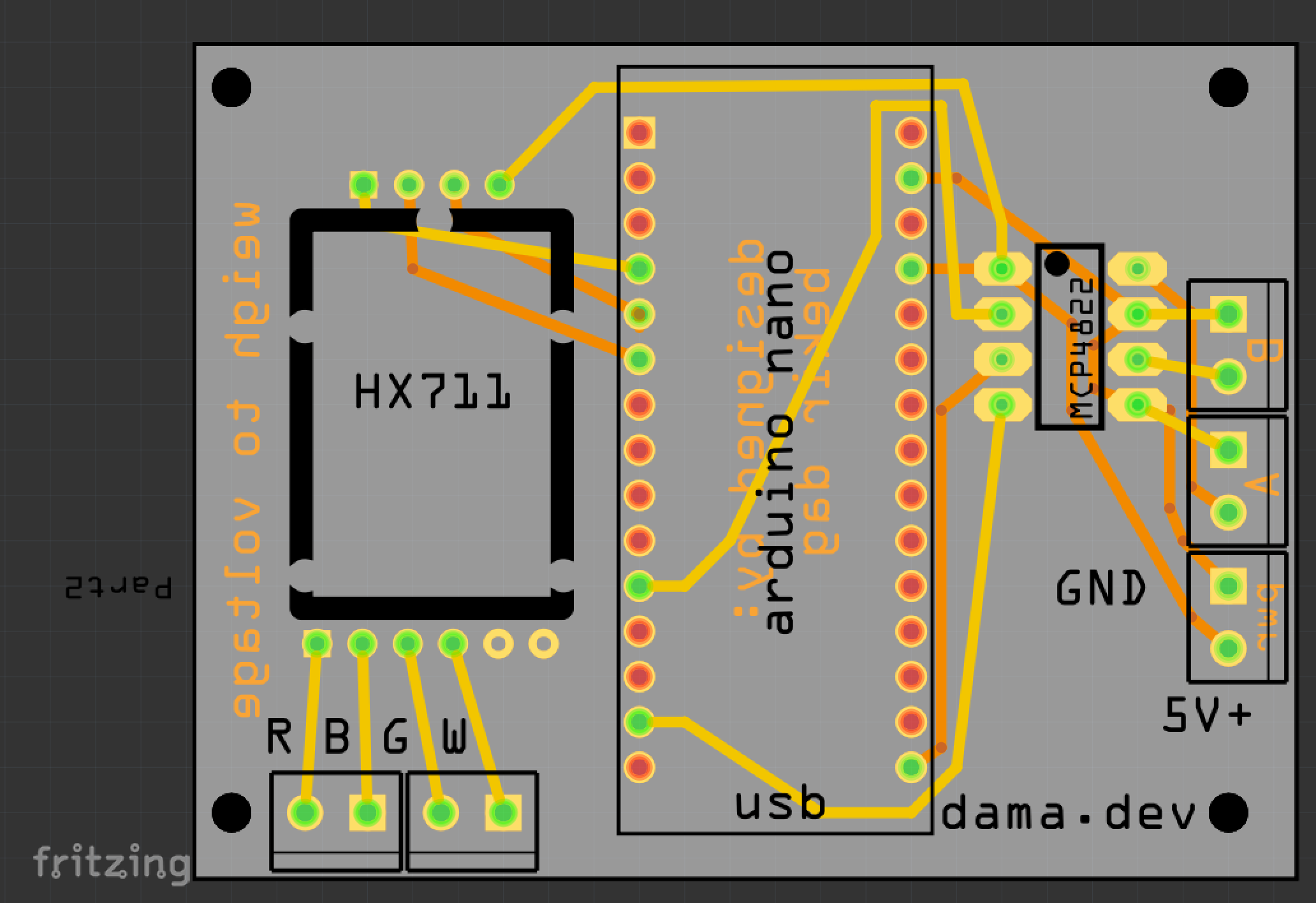They sent it pretty fast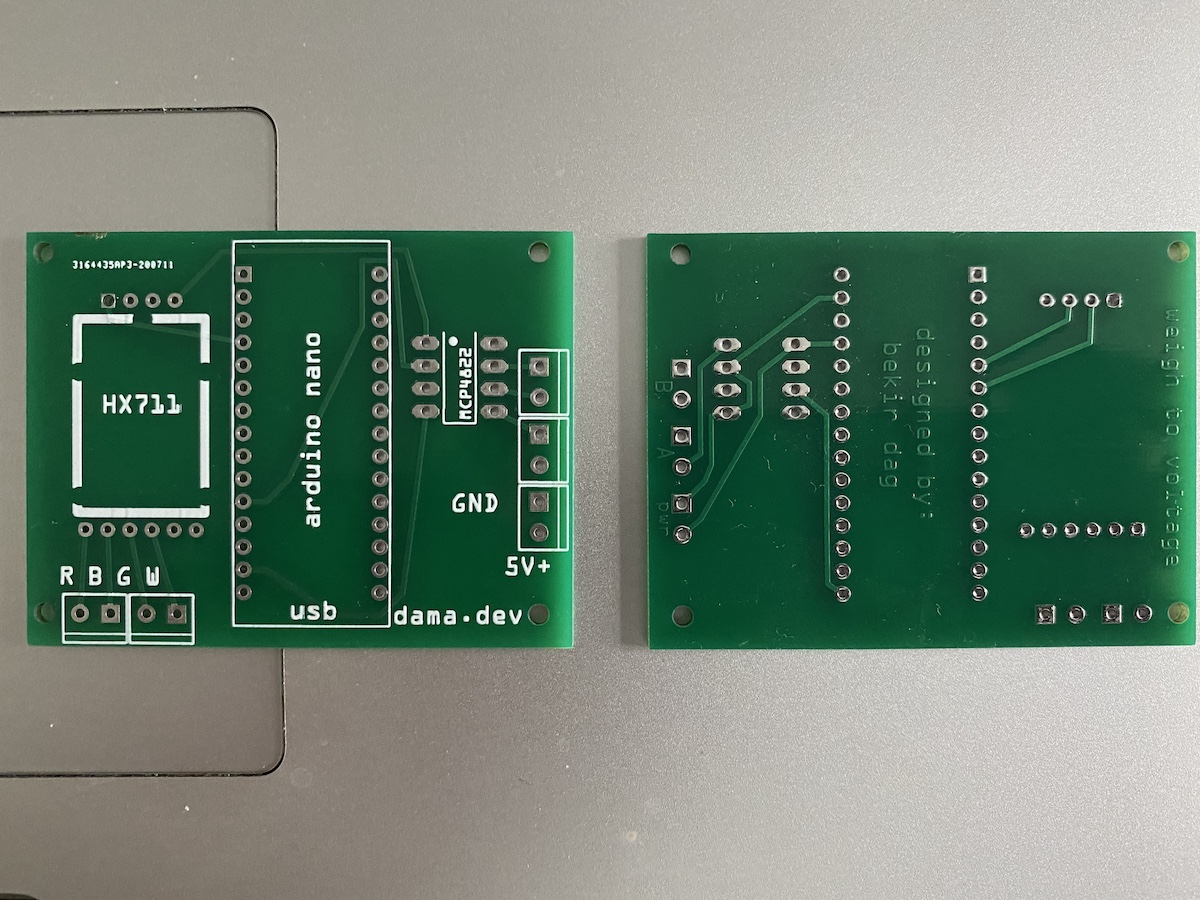I will share the code here as well, so you can use the same

/*
D. Mohamad 29/03/17 code to commumincate with MCP4822 12-bit DAC via SPI
pin assignments as follows...
Uno (master)  MCP4822 (slave)
CS    8             2
MOSI  11            4
SCK   13            3
Read output voltage with a multimeter Va/Vb on pin 8/6.
send commands via serial interface eg.
AL1000 would mean...
channel=A
gain=low
D=1000
Va = gain*Vref*D/4095
= 1*2.04*1000/4095 = 0.498V
see www.theonlineshed.com
*/

/* you need to send a message like ah100 in serial monitoring to test */

#include <SPI.h>
#include "HX711.h"

const int DAC_CS = 8; //Chip select pin for the DAC
String inputString = ""; //holds serial commands

// HX711 circuit wiring

int can_weight = 0;
int min_weight = 0;
int max_weight = 650;
int min_milivolts = 0;
int max_milivolts = 4096;
int output_voltage = 0;

int weight_to_voltage_ratio = (max_milivolts-min_milivolts)/(max_weight-min_weight);

HX711 scale;

void setup() {
// set the CS as an output:
pinMode (DAC_CS, OUTPUT);
Serial.begin(9600);     // opens serial port, sets data rate to 9600 bps
inputString.reserve(200); // reserve 200 bytes for the inputString:
SPI.begin();

Serial.println("Initializing the scale");

// Initialize library with data output pin, clock input pin and gain factor.
// Channel selection is made by passing the appropriate gain:
// - With a gain factor of 64 or 128, channel A is selected
// - With a gain factor of 32, channel B is selected
// By omitting the gain factor parameter, the library
// default "128" (Channel A) is used here.

Serial.println("Before setting up the scale:");

Serial.print("get value: \t\t");
Serial.println(scale.get_value(5));   // print the average of 5 readings from the ADC minus the tare weight (not set yet)

Serial.print("get units: \t\t");
Serial.println(scale.get_units(5), 1);  // print the average of 5 readings from the ADC minus tare weight (not set) divided
// by the SCALE parameter (not set yet)

scale.set_scale(856.f);     // CHANGE THIS VALUE TO CALIBRATE! this value is obtained by calibrating the scale with known weights; see the README for details
scale.tare();               // reset the scale to 0

Serial.println("After setting up the scale:");

Serial.print("get value: \t\t");
Serial.println(scale.get_value(5));   // print the average of 5 readings from the ADC minus the tare weight, set with tare()

Serial.print("get units: \t\t");
Serial.println(scale.get_units(5), 1);        // print the average of 5 readings from the ADC minus tare weight, divided
// by the SCALE parameter set with set_scale

}
// lets convert the weigh into milivolts. it should be around 0mV - 4096mV. The weigh range is 0 - 650g.
void loop() {

unsigned int n; //number 2 bytes long unsigned
char channel, gain;
String errmsg;  //errors returned from DAC_set

Serial.print(scale.get_units(), 1);
Serial.print("\t| average:\t");
Serial.println(scale.get_units(3), 1);

can_weight = scale.get_units(3);

output_voltage = weight_to_voltage_ratio*can_weight;
channel = "a";
gain = "h";
errmsg="";

DAC_set(output_voltage, channel, gain, DAC_CS, errmsg);

scale.power_down();              // put the ADC in sleep mode
delay(50);
scale.power_up();

//only run when data is available
if (Serial.available() > 0){
serial_DAC_set();
}
}

// just use this function to set the voltage on either channel A or B
//function to set state of DAC - input value between 0-4095
void DAC_set(unsigned int input, char DAC_sel, char Gain_sel, int CS_pin, String &errmsg)
{
//DAC_sel choose which DAC channel you want to write to A or B
//Gain_sel choose your gain: H=2xVref and L=1xVref
byte MSB,LSB;//most sig, least sig bytes and config info

//clear error messages
errmsg="";

Serial.flush();  // in case of garbage serial data, flush the buffer
delay(10);

//only run the rest of the code if binary is in range.
if (input<0 || input >4095)
errmsg += "input out of range. 0-4095.";
else
{
//convert decimal input to binary stored in two bytes
MSB = (input >> 8) & 0xFF;  //most sig byte
LSB = input & 0xFF;         //least sig byte

//apply config bits to the front of MSB
if (DAC_sel=='a' || DAC_sel=='A')
MSB &= 0x7F; //writing a 0 to bit 7.
else if (DAC_sel=='b' || DAC_sel=='B')
MSB |= 0x80; //writing a 1 to bit 7.
else
errmsg += "DAC selection out of range. input A or B.";

if (Gain_sel=='l' || Gain_sel=='L')
MSB |= 0x20;
else if (Gain_sel=='h' || Gain_sel=='H')
MSB &= 0x1F;
else
errmsg += "Gain selection out of range. input H or L.";

//get out of shutdown mode to active state
MSB |= 0x10;

Serial.println("binary input to DAC: ");
Serial.print(MSB,BIN);
Serial.print(" ");
Serial.println(LSB,BIN);

//now write to DAC
// take the CS pin low to select the chip:
digitalWrite(CS_pin,LOW);
delay(10);
//  send in the address and value via SPI:
SPI.transfer(MSB);
SPI.transfer(LSB);
delay(10);
// take the CS pin high to de-select the chip:
digitalWrite(CS_pin,HIGH);
}
}

//function to read user command from the serial command window and set the DAC output
void serial_DAC_set(void)
{
unsigned int n; //number 2 bytes long unsigned
char channel, gain;
String errmsg;  //errors returned from DAC_set

// say what you got:
Serial.println(inputString);

channel = inputString.charAt(0);
//and gain
gain = inputString.charAt(1);
//convert string to an int - binary voltage
n = inputString.substring(2).toInt();
//set DAC state
DAC_set(n, channel, gain, DAC_CS, errmsg);

//print errors if there are any

Serial.print("n: ");
Serial.println(n);
Serial.print("channel: ");
Serial.println(channel);
Serial.print("gain: ");
Serial.println(gain);
Serial.print("DAC_CS: ");
Serial.println(DAC_CS);
Serial.print("errmsg: ");
Serial.println(errmsg);

//clear input string ready for the next command
inputString = "";
}